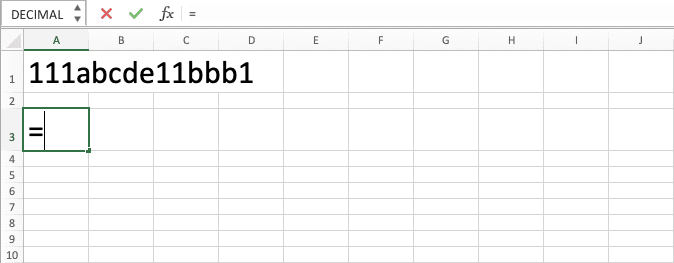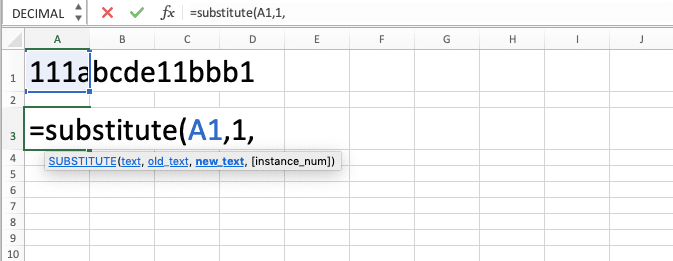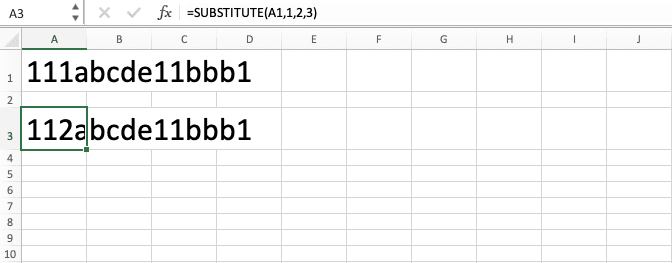How to Use the SUBSTITUTE Formula in Excel: Functions, Examples, and Writing Steps - Compute Expert

# How to Use the SUBSTITUTE Formula in Excel: Functions, Examples, and Writing Steps

Home >> Excel Tutorials from Compute Expert >> Excel Formulas List >> How to Use the SUBSTITUTE Formula in Excel: Functions, Examples, and Writing Steps

In this tutorial, you will learn how to use the SUBSTITUTE formula in excel completely.

When working with our data in excel, we sometimes want to replace part of our data with something else. One of the easiest ways to do that is by using this excel SUBSTITUTE formula.

Want to know more about SUBSTITUTE and how to use it properly in excel? Read this tutorial until its last part!

Disclaimer: This post may contain affiliate links from which we earn commission from qualifying purchases/actions at no additional cost for you. Learn more

## What is the SUBSTITUTE Formula in Excel?

SUBSTITUTE is a formula that helps you to replace some parts of your data with another thing you want.

## SUBSTITUTE Function in Excel

You can use SUBSTITUTE to replace an instance or all instances of your data part with something else.

## SUBSTITUTE Result

The SUBSTITUTE result is data that you have replaced its part of with something else.

## Excel Version from Which You Can Start Using SUBSTITUTE

You can start using SUBSTITUTE in excel since excel 2003.

## The Way to Write It and Its Inputs

Here is the general writing form of SUBSTITUTE in excel.

= SUBSTITUTE ( data , data_part_to_replace , data_part_replacement , [ data_part_instance_to_replace ] )

And here is a bit explanation of the inputs you need to give to SUBSTITUTE.
• data = the data which you want to replace its part of
• data_part_to_replace = the part of the data you want to replace with something else
• data_part_replacement = the replacement you want for the part of the data
• [data_part_instance_to_replace] = the instance of the data part you want to replace in the data. If you omit this input, SUBSTITUTE will replace all the instances of the data part

## Example of Its Usage and Result

Here is an implementation example of SUBSTITUTE in excel.In this example, we use SUBSTITUTE to replace parts of all the data that we have. You can see how we write SUBSTITUTE on the most right there to get the results on their left.

With SUBSTITUTE, you can also remove data parts as you can see in the fifth row of the example. To do that, just input a blank cell or empty quotes (“”) in your SUBSTITUTE data part replacement input part.

## Writing Steps

Need some guidance when you write your SUBSTITUTE formula in excel? Here is a step-by-step one that you can use.

1. Type an equal sign ( = ) in the cell where you want to put the result in2. Type SUBSTITUTE (can be with large and small letters) and an open bracket sign after =3. Input the data you want to change the part of. Then, type a comma sign ( , )4. Input the data part you want to change from the data. Then, type a comma sign5. Input the replacement you want for the data part6. Optional: Type a comma sign. Then, input a number that represents the instance of the data part you want to replace. If there is no input here, SUBSTITUTE will replace all the instances of the data part you want to replace7. Type a close bracket sign8. Press Enter
9. Done!

Result with the optional input that represents the data part instanceResult without the optional input that represents the data part instance## SUBSTITUTE vs REPLACE

There is also another formula you can use in excel to replace a part of your data. That formula name is REPLACE. Now, what is the difference between this REPLACE formula and SUBSTITUTE, and which one should we use?

First of all, let’s take a look at how we generally write REPLACE in excel.

= REPLACE ( data , position_of_data_part_to_replace , number_of_characters_of_the_data_part_to_replace , data_part_replacement )

You might notice that the REPLACE inputs are a bit different to SUBSTITUTE. If you use REPLACE, you need to input the position of the data part you want to replace in your data (position 1 is the most left position in the data). You also need to input the number of characters of the data part you want to replace.

Meanwhile, SUBSTITUTE requires you to input the data part you want to replace directly. You can also input the instance you want to replace or it will replace all the data part instances.

Thus, that is the key difference you should remember when you choose between using SUBSTITUTE or REPLACE. If you want to have a say in the data part position to replace, then you should use REPLACE. However, if you want to replace the data part based on instances, you should use SUBSTITUTE instead!

Here is an implementation example of REPLACE in excel to make you understand better about the formula.You can see in the example how we use REPLACE in excel. Instead of inputting the data part we want to replace directly, we input its position and number of characters.

Consider the inputs you need to give when you use SUBSTITUTE or REPLACE and utilize the best one for your situation!

## Nested SUBSTITUTE

If you need to replace different data parts in your data at once in excel, you can use nested SUBSTITUTE.

Here is the general writing form of the nested SUBSTITUTE in excel to serve the purpose.

= SUBSTITUTE ( SUBSTITUTE ( … , data_part_to_replace1 , data_part_replacement1 , [ data_part_instance_to_replace1 ] , data_part_to_replace2 , data_part_replacement2 , data_part_instance_to_replace2 ] ) )

Nest your SUBSTITUTE in the data input part of the previous SUBSTITUTE. Write SUBSTITUTEs as many as the number of different data parts you want to replace in your data.

Here is the implementation example of the nested SUBSTITUTE in excel to make you understand the concept easier.In this example, we try to replace our month abbreviations with what they stand for. Because we have multiple abbreviations here in one data, we use nested SUBSTITUTE.

As we have three months’ abbreviations we want to replace, we nest three SUBSTITUTE here. We input each month’s abbreviation with what it stands for in each SUBSTITUTE.

As a result, we get what we want in the data that our nested SUBSTITUTE produces!

## Exercise

After you have learned how to use SUBSTITUTE in excel, now let’s deepen your understanding by doing the following exercise!

Download the exercise file and do all the instructions! Download the answer key file if you have done the exercise and want to check your answers. Or probably if you are confused and want some clues on how to do the instructions!

### Instructions

Use SUBSTITUTE to do all the instructions below!
1. Replace “a” letters in the left code with “c”!
2. Replace all 1 in the left code with 0!
3. Replace the second “x” letter in the left code with “z”!

SUBSTITUTE is case-sensitive and doesn’t recognize wildcard characters input.

Related tutorials you should learn from:

Get updated excel info from Compute Expert by registering your email. It's free!